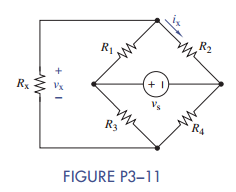### Create an Account

Already have account?

### Forgot Your Password ?

Home / Questions / Formulate node-voltage equations for the circuit in Figure P3 11 b Solve for vx and ix when

# Formulate node-voltage equations for the circuit in Figure P3 11 b Solve for vx and ix when

(a) Formulate node-voltage equations for the circuit in Figure P3−11.

(b) Solve for vx and ix when R1 =1kΩ, R2 = 1:5 kΩ, R3 = 500 Ω, R4 =2kΩ, Rx = 100 Ω, and v= 15 V.Jun 15 2020 View more View Less

#### Answer (Solved)Subscribe To Get Solution